# Learn how to solve a Skewb - Notations Guide | KewbzUK

by Luke Terzich

### Want to learn the Skewb Notation?

Want to learn the correct notation for the Skewb Puzzle? Look no further than KewbzUK.  In this guide we will look at the correct notations for the Skewb.

We will go through 2 different notations based on the method you are using.

### Beginners Method Notation

The Beginners method uses a different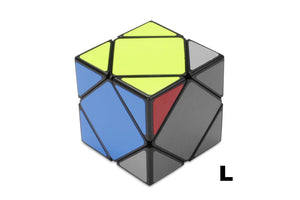The Beginners method for the Skewb uses the above rotation for the 'L' move.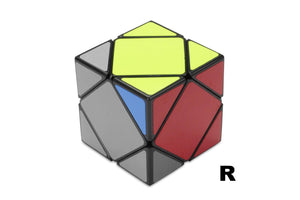The Beginners method for the Skewb uses the above rotation for the 'R' move.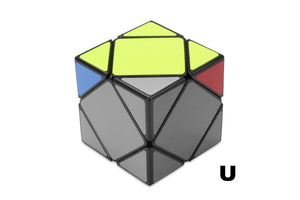With 'U' moves in the Skewb Notation, simply move the part of the Skewb as shown above.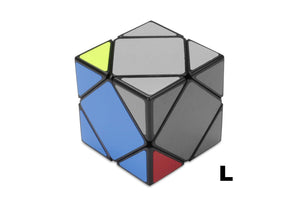With 'L' moves in the Skewb Notation, simply move the part of the Skewb as shown above.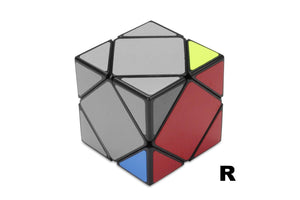With 'R' moves in the Skewb Notation, simply move the part of the Skewb as shown above.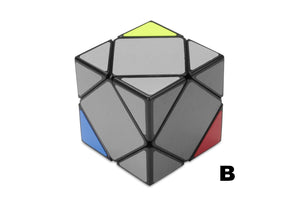With 'B' moves in the Skewb Notation, simply move the part of the Skewb as shown above.